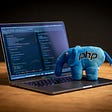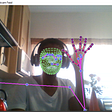# Uber and Arrays

This post is about a very beautiful interview question that was once asked by Uber. Question statement is as follows:

Given an array of integers, return a new array such that each element at index i of the new array is the product of all the numbers in the original array except the one at i.

For example, if our input was [1, 2, 3, 4, 5], the expected output would be [120, 60, 40, 30, 24]. If our input was [3, 2, 1], the expected output would be [2, 3, 6].

The easiest approach somebody can think of is, for any array element at index i, take the product of all the other elements of the array excluding the current index and store the product for current index i.e. index i in a product array at it’s index i.

This is a very basic and naive approach and would result in a big time complexity as we have to traverse the array n times for filling it, then for each element again traverse n-1 elements to compute product. It means a time complexity of order of O(n)+O(n*(n-1)).

We need to think of a better approach that can do the same task in less time. So, what if we use the technique of multiplication and division together.

Sounds tricky! We together will make it simple. Let’s do it.

Suppose we have an array of non zero integers, A=[1,2,3,4] and we have to find it’s product array, say P=[]. We first declare a variable named product and initialize it to a value of 1. Now while filling the array A, we calculate the product as:

for i=0 to A.length()-1:

product= product * A[i];

(considering 0-based indexing)

product in our case will be 1*2*3*4= 24.

Now we have reached our final step of populating the product array, P. We initialize elements of P by traversing the product array from index i=0 to i=A.length()-1 as:

P[i]=product/A[i];

This ensures that any element at index i of P[], will have the product of remaining elements except the element itself. Our P becomes, [24,12,8,6].

Are we done? For some people we might be done. But in reality, we aren’t! So, what are we left with?

If you look closely, we have only considered the case when array have non-zero values. What if the array have zero values as well? Well for that you don’t need to worry much. I will show you! With a little trick in the above technique, we can handle the zero case.

Consider the array, A=[1,2,0,5]

The output product array should be, P=[0,0,10,0]. But with the above code, we definitely can’t achieve that. The changes we need to make are:

While traversing the array A first time, we need to keep count of three things:

• Non zero product (product)
• If there is a zero element (isZero)
• Number of zeros (numZeros)

Non zero product is product of all Ai’s that are not zero.

While traversing A, if we find a zero element, then set the Boolean true flag for ifZero variable.

Count the number of zeros in A and store it into numZeros variable.

Now, when we are done with this, we need to populate our product array, i.e. P.

For that we first check if numZeros>1.

• If numZeros is greater than 1, that means the original array has at least two zeros and therefore the product array will contain all zeros and we are done. A=[1,2,0,0], therefore P=[0,0,0,0]
• If numZeros is less than or equal to 1, this means that the original array A must be having either one zero or no zero. If isZero is false, it becomes a case of all non zero values in the array can be solved easily (the approach we discussed in the post above). But if isZero is true, that means the array have one element as zero. Then in that case, to populate the product array P, we have to take special care when A[i] is zero. It’s not complicated and we can easily go about that. We can simply populate the product array this way:

If(A[i]) P[i]=0;

else P[i]=product;

Here, if the current element is non zero then it’s product counterpart should be zero. If the current element is zero, it’s product counterpart should be non zero.

And now, we are finally done! This way we have reduced the time complexity to O(n)+O(n). O(n) for traversing the array A first time and O(n) for populating the product array. This is all for this post.

I hope you guys enjoyed the post.

If you find it helpful, please share and claps are definitely appreciated! ☺

Now comes a teaser, what if you can’t use division? Well, I will soon write a post on the same. Till then, Feel free to comment, if you have any queries.

--

--

--

## More from TRICK THE INTERVIEWER

Programming interview is the one where the interviewer will make all possible attempts to trick you. Trick the interviewer this time! :)

## Statement Conditional — Python## Docker for beginners## Email Syntax Validation and Existence Verification in PHP## My First TDD Code Kata## Artificial Intelligence Project: Pose Detection## Design Patterns In Project — Template & Strategy## I will personally use this time before SynAssets to acquire or work toward getting SynAssets…## Jhanak Didwania

Software Engineer | Programmer

## Ramlal’s Problem — Understanding System Design## Why are design patterns classified into just 3 categories?## Top Leetcode Browser Extensions## What is the difference between Strategy, State, and Template design patterns?